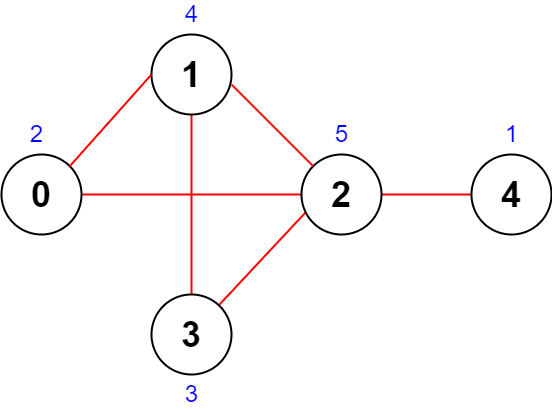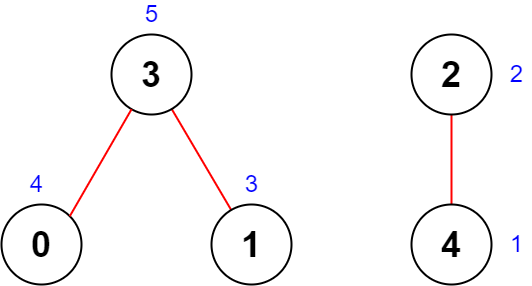##### Welcome to Subscribe On Youtube

Formatted question description: https://leetcode.ca/all/2285.html

# 2285. Maximum Total Importance of Roads

• Difficulty: Medium.
• Related Topics: Greedy, Graph, Sorting, Heap (Priority Queue).
• Similar Questions: .

## Problem

You are given an integer n denoting the number of cities in a country. The cities are numbered from 0 to n - 1.

You are also given a 2D integer array roads where roads[i] = [ai, bi] denotes that there exists a bidirectional road connecting cities ai and bi.

You need to assign each city with an integer value from 1 to n, where each value can only be used once. The importance of a road is then defined as the sum of the values of the two cities it connects.

Return the **maximum total importance of all roads possible after assigning the values optimally.**

Example 1:Input: n = 5, roads = [[0,1],[1,2],[2,3],[0,2],[1,3],[2,4]]
Output: 43
Explanation: The figure above shows the country and the assigned values of [2,4,5,3,1].
- The road (0,1) has an importance of 2 + 4 = 6.
- The road (1,2) has an importance of 4 + 5 = 9.
- The road (2,3) has an importance of 5 + 3 = 8.
- The road (0,2) has an importance of 2 + 5 = 7.
- The road (1,3) has an importance of 4 + 3 = 7.
- The road (2,4) has an importance of 5 + 1 = 6.
The total importance of all roads is 6 + 9 + 8 + 7 + 7 + 6 = 43.
It can be shown that we cannot obtain a greater total importance than 43.


Example 2:Input: n = 5, roads = [[0,3],[2,4],[1,3]]
Output: 20
Explanation: The figure above shows the country and the assigned values of [4,3,2,5,1].
- The road (0,3) has an importance of 4 + 5 = 9.
- The road (2,4) has an importance of 2 + 1 = 3.
- The road (1,3) has an importance of 3 + 5 = 8.
The total importance of all roads is 9 + 3 + 8 = 20.
It can be shown that we cannot obtain a greater total importance than 20.


Constraints:

• 2 <= n <= 5 * 104

• 1 <= roads.length <= 5 * 104

• roads[i].length == 2

• 0 <= ai, bi <= n - 1

• ai != bi

• There are no duplicate roads.

## Solution (Java, C++, Python)

• class Solution {
public long maximumImportance(int n, int[][] roads) {
int[] degree = new int[n];
int maxdegree = 0;
for (int[] r : roads) {
degree[r]++;
degree[r]++;
maxdegree = Math.max(maxdegree, Math.max(degree[r], degree[r]));
}
Map<Integer, Integer> rank = new HashMap<>();
int i = n;
while (i > 0) {
for (int j = 0; j < n; j++) {
if (degree[j] == maxdegree) {
rank.put(j, i--);
degree[j] = Integer.MIN_VALUE;
}
}
maxdegree = 0;
for (int d : degree) {
maxdegree = Math.max(maxdegree, d);
}
}
long res = 0;
for (int[] r : roads) {
res += rank.get(r) + rank.get(r);
}
return res;
}
}

• Todo

• class Solution:
def maximumImportance(self, n: int, roads: List[List[int]]) -> int:
deg =  * n
deg[a] += 1
deg[b] += 1
deg.sort()
return sum(i * v for i, v in enumerate(deg, 1))

############

# 2285. Maximum Total Importance of Roads

class Solution:
def maximumImportance(self, n: int, roads: List[List[int]]) -> int:
count = defaultdict(int)
​
count[a] += 1
count[b] += 1
​
scores = defaultdict(int)

v = sorted(count.items(), key = lambda x:-x)

for k, cnt in v:
scores[k] = n
n -= 1

res = 0

res += scores[a] + scores[b]

return res



Explain:

nope.

Complexity:

• Time complexity : O(n).
• Space complexity : O(n).